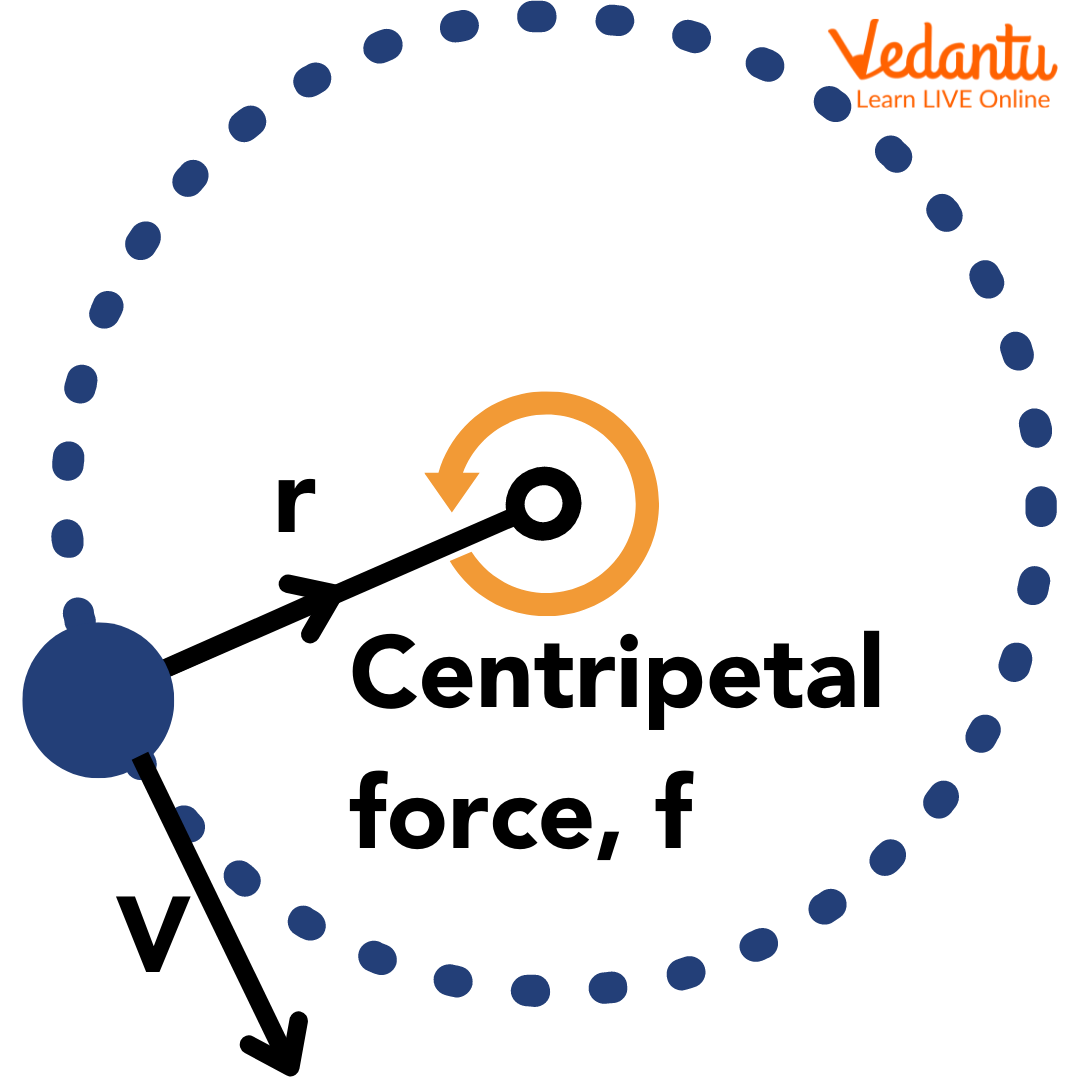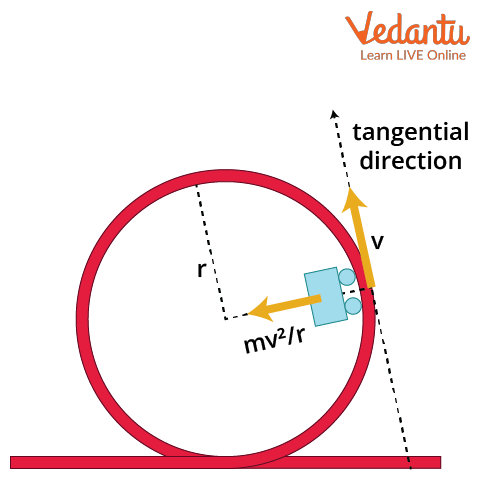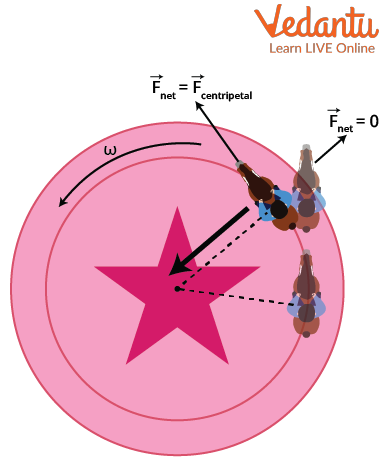Courses
Courses for Kids
Free study material
Free LIVE classes
More

# Centripetal Force - Important Concepts with Formula based Examples for JEE## What is Centripetal Force?

Last updated date: 26th Mar 2023
Total views: 76.5k
Views today: 1.53k

What is centripetal force? The centripetal force can be defined as any motion in a curved path that represents accelerated motion and necessitates a force directed toward the path's centre of curvature. This force is known as the centripetal force, which is also known as the "centre seeking" force. The centripetal force is always perpendicular to the direction of the object's displacement. The centripetal force direction for an object moving in a circular path is always directed towards the centre of the circle, according to Newton's second law of motion. For example, the Sun's gravitational force is a centripetal force that helps the Earth and keeps the Earth orbiting it. The unit of centripetal force is Newton (N).

This article will include the basics of the centripetal force, its formula, examples, characteristics, and the work done by the centripetal force. This article will cover all about the centripetal force.

## Centripetal Force Formula

When an object travels in the circular path with the constant speed, it experiences an accelerating centripetal force towards the centre.

The equation for the centripetal force is as shown below:

$F=\dfrac{m v^{2}}{r}$

where

m is the mass of the object,

v represents the velocity or speed of the object, and

Hence, this is the centripetal force equation.

## Dimensions of Centripetal Force

Dimensional formula for the centripetal force can be given as:

$F=\dfrac{m v^{2}}{r}$

The dimensions of velocity is LT-1

Dimensions of mass, M

Dimensions of force can be calculated as

Power of M=1

Power of v=2

Power of r=−1

So, the dimensions of centripetal force is MLT-2

## Work Done by Centripetal ForceImage 1: Centripetal  Force Diagram

Work done is the dot product of the force applied to an object and its displacement.

W = F.S

$=F\cdot S\cos{\theta}$

$\theta$ is the angle between displacement and force.

In the case of centripetal force, the force is always directed towards the centre of the circular path. As the force acts perpendicular to the motion and not in the same direction, so the work done by the centripetal force is given by the following equation as:

W=FS(cos 90) =0

## Centripetal Force Examples

• The Roller coaster’s track has sharp turns as well as it is curved. The seat or wall pushes you towards the centre when you ride on a roller coaster, but the normal force provides the centripetal force and keeps you along the curved track.Image 2: A Roller Coaster Track

• A merry-go-round is nothing more than a spinning disc. The child sitting on the disc is at rest, but he is moving due to the circular motion of the disc. The support on the disc provides children with centripetal force, causing them to be on a moving disc.Image 3: A Merry-go-round

• Centripetal force is used in medical centrifuges to accelerate the precipitation of suspended particles in the blood. The use of a centrifuge to accelerate a blood sample (600 to 2000 times its normal gravity acceleration) prevents blood cells from settling with the rest of the blood sample. The heavier red blood cells will sink to the bottom of the tube, while the other components will settle in layers according to their densities. As a result, it is now possible to easily separate blood cells and other components.Image 4: Centrifuge Machine

• The centripetal force between your clothes and the interior of the drum pushes them around in a circle. There's nothing to give it the same kind of shove because the water can pass right through the drum holes. The clothing experiences centripetal force, but the water does not. While the garments spin in a circle, the water flows straight through the perforations. And that is how you dry your clothes.Image 5: Washing Machine Dryer

### Characteristics of Centripetal Force

Characteristics of centripetal force are listed below:

• Centripetal force is provided by gravitational force, frictional force, electromagnetic force, etc. and is the real force.

• Objects move in a circle due to the centripetal force.

• It points in the direction of the centre of the circular motion.

• The sense of rotation of the body has no impact on the direction of centripetal force.

## Solved Example

Example: A ball is attached to a string that is 1.5m long. What is the centripetal acceleration felt by the ball if it is spun so that it completes two full rotations every second?

Ans: To find the centripetal acceleration i.e. written as

$a=\dfrac{v^2}{r}$

We have the radius given in the question as the circle with the radius of the string as 1.5m and it also completes two full rotations per second. As it is moving in a circle, so the length of each rotation will be the circumference of the circle that will be given by:

\begin{align} &C=2 \pi r \\ &C=2 \pi(1.5) \\ &C=3 \pi \end{align}

The velocity of the ball can be found by multiplying the frequency and the distance covered by the ball as:

\begin{align} v=C f \\ &v=3 \pi(2) \\ & v=6 \pi \end{align}

So, the acceleration will be given as:

\begin{align} &a=\dfrac{v^{2}}{r} \\ &a=\dfrac{(6 \pi)^{2}}{3 \pi} \\ &a=12 \pi \dfrac{\mathrm{m}}{ \mathrm{s}^{2}} \end{align}

## Summary

In short, centripetal force is a force acting on a body moving in a circle and directed towards the centre around which the body is moving. If the particles decrease or increase their speed during the circular motion, the resultant force deflects the particle from its original path. We have discussed the centripetal force, its formula, examples, characteristics, and the various factors that help us to understand the topic clearly.

## FAQs on Centripetal Force - Important Concepts with Formula based Examples for JEE

1. What is Centripetal Acceleration?

Centripetal Acceleration should be defined as follows :

centripetal acceleration is the property of motion of an object navigating a circular path. Centripetal acceleration is based on the idea that when an object moves in a circular motion, its acceleration vector will point to the centre of the circle. Even if the object is moving at a constant speed, this phenomenon will occur. There is a similarity between centripetal acceleration and centripetal force that they both have the same direction.

2. What are the factors affecting centripetal force?

Some of the important factors that will affect the centripetal force are given below:

(i) Mass of the object (m) - as from the centripetal force formula, we can see that

force is directly proportional to the mass of the object.

(ii) Velocity of the object (v) - as from the centripetal force formula, we can see that

force is directly proportional to the square of the velocity.

(iii) The radius of the circle (r) - as from the centripetal force formula, we can see that

force is inversely proportional to the square of the velocity.